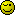# Need help with these questions

-Write a function that takes 1 parameter and writes the squared value of that parameter to the page.

-Write a function that gets the value from a HTML Input element named “numberInput”, divides it by 3 and writes the value to the page.

These are some questions in my intro coding class, just need help asnwering them/

Hi there kestrin15,

and a warm welcome to these forums.1. Do you understand the questions?
2. If you do, can we see your attempts at answering them?
3. If you don’t, do you want us to write code that you
probably won’t be able understand at your present level?

Yeah, it’s not a test question or anything. I like learning from seeing the code typed out, I can learn from looking at the answers(code) and figuring it out! So go ahead and write it out and I will let you know if I am confused

“it’s not a test question or anything”. Well now that you’ve said thatYour intro class has introduced functions, correct?

What part of the questions are you having trouble with?
Do you understand what a function is? Do you know how to write one?
Do you understand what a parameter is?
Do you understand how Javascript interacts with DOM elements? Do you understand what a DOM element is?

1 Like

Here you go…

``````<!DOCTYPE HTML>
<html lang="en">

<meta charset="utf-8">
<meta name="viewport" content="width=device-width,height=device-height,initial-scale=1">

<title>untitled document</title>

<style media="screen">
body {
background-color: #f0f0f0;
font: 1em/150% verdana, arial, helvetica, sans-serif;
text-align: center;
}

h1 {
font-size: 1em;
}

.number-testing {
display: inline-block;
border: 0.062em solid #999;
box-shadow: 0.4em 0.4em 0.4em rgba( 0, 0, 0, 0.3 );
background-color: #fff;
text-align: left;
}

.number-testing div {
}

.number-testing label{
display: block;
}
</style>

<body>

<h1>Number manipulation</h1>

<form class="number-testing">
<div>
<label for="base0">base number to square: </label>
<input id="base0" type="number" value="0">
<label for="squared">base number squared: </label>
</div><div>
<label for="base1">base number to divide by 3: </label>
<input id="base1" type="number"  value="0">
<label for="divided">base number divided by 3: </label>
</div><div>
<input type="reset" value="clear fields">
</div>
</form>

<script>
(function( d ) {
'use strict';

d.getElementById( 'base0' ).addEventListener( 'input', function() {
d.getElementById( 'squared' ).value = this.value * this.value;
}, false );

d.getElementById( 'base1' ).addEventListener( 'input', function() {
d.getElementById( 'divided' ).value = this.value / 3;
}, false );
}(  document ));
</script>

</body>
</html>
``````

``````const squared = x => x*x;
``````const showNumberInput = form => {